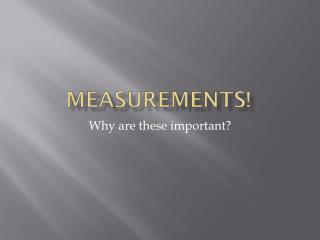DownloadDownload PresentationMeasurements!

# Measurements!

Télécharger la présentation## Measurements!

- - - - - - - - - - - - - - - - - - - - - - - - - - - E N D - - - - - - - - - - - - - - - - - - - - - - - - - - -
##### Presentation Transcript

1. Measurements! Why are these important?

2. SI • SI stands for Systeme International d’Unites • Internationally, this is the system that we will use measure anything in science. • There are several things that get measured • Length, Mass, Volume/Area, Density, Time, Temperature

3. Length • Measured in Meters (m) • How long something is or the distance between two objects

4. Mass • Measured in grams (g) • The amount of matter in an object • Not the same as weight but similar

5. Area/Volume • Area is measured in m2 • It is the amount of space on the surface something takes up. • Volume is measured in the liter (L) or m3 • Amout of space something takes in 3D

6. Measuring a Liquid’s Volume • When measuring from a graduated cylinder or a beaker, measure at the bottom of the meniscus (natural curve that water makes in a container)

7. Density • Amount of matter that occupies a given space • Doesn’t have a special unit to itself. • Labeled g/mL or g/cm3 • Aka mass/volume • No tool to measure

8. Time • Internationally time is measured by seconds (s) • Usually is going to be measured by a watch or a timer

9. Temperature • In science we do no use the Fahrenheit scale. We use Celcius (C°) • We could also measure in Kelvin but that’s less important at the moment • Use a thermometer

10. Unit • Unit is whatever you are measuring in. It is described as any of the SI measurements that are used • Denoted with a u

11. Goes by 10’s • There are bigger and smaller units of each base units • Length-meter(m) • Mass- gram (g) • Volume- Liter (L) • Time- second (s) • Temperature- Celcius (C°) • Prefixes will go in front of the base unit. • Prefixes are: kilo-, hecto-, deka-, deci-, centi-, milli-, micro-, nano-, pico- • King Henry Doesn’t [Usually] Drink Chocolate Milk

12. Goes by 10’s • Kilo(k)- kilogram (kg), kilometer (km), kiloliter (kL), kilosecond (ks), kilounit (ku) 1ku= 1000 u (1000 of your base unit) • 1u= .001 ku • Hecto(h)- hectogram (hg), hectometer (hm), hectoliter (hL), hectosecond (hs), hectounit (hu) 1 hu= 100 u (100 of your base unit) • 1 u= .01 hu

13. Goes by 10’s • Deka(D)- dekagram (Dg), dekameter (Dm), dekaliter (DL), dekasecond (Ds), dekaunit (Du) • 1Du= 10 u (10 of your base unit) • 1 u= .1 Du

14. Goes by 10’s • Deci(d)- decigram (dg), decimeter (dm), deciliter (dL), decisecond (ds), deciunit (du) • 1 u= 10 du (10 du in your base unit) • 1du = .1u • Centi (c)- centigram (cg), centimeter (cm), centiliter (cL), centisecond (cs), centiunit (cu) • 1 u= 100 cu (100 cu in your base unit) • 1cu= .01 u

15. Goes by 10’s • Milli (m)- milligram (mg), millimeter (mm), milliliter (mL), millisecond (ms), milliunit (mu) • 1 u= 1000 mu (1000 mu in your base unit) • 1 mu= .001 u • Quick Review….

16. Practice • 1 m = ? mm • 1ms= ? s • 1 L = ? cL = ? mL • 1 L = ? mm • HINT! • If you are getting bigger divide. • If you are getting smaller multiply

17. Measurement Uncertainty • Accuracy- is a measure of how close a measurement comes to the actual value of whatever is measured. • Ex. Darts land close to the bull’s-eye

18. Measurement Uncertainty • Precision- the measure of how close a series of measurements are to one another • Ex. The darts are all very closely grouped together. (Don’t have to be near bull’s-eye) • If something is precise it isn’t always accurate.

19. Questions • The density of a certain metal is 2.45 g/mL. • You measure densities of 2.01 g/mL, 1.99 g/mL and 2.03 g/mL. Accurate, precise, or both? • You measure densities of 2.45 g/mL, 2.50 g/mL, and 2.47 g/mL. Accurate, precise, or both? • You measure densities of 1.57 g/mL, 3.01 g/mL, and 2.45 g/mL

20. Finding Error • Accepted value- The correct value based on reliable references • Experimental value- The value measured in the lab

21. Finding Error • Error is the difference between the experimental value and the accepted value. • Error= experimental value- accepted value • The density of gold is 19.30 g/mL. You do a experiment and find a density for gold of 20.50 g/mL. What is the error in your experiment?

22. Finding Error • Error can be positive or negative depending on whether the experimental value is great than or less than the accepted value. • Percent error is the absolute value of the error divided by the accepted value, multiplied by 100%. • Percent error= error/(accepted value) x 100% • Gold PE = 1.20(g/mL)/19.30(g/mL)x 100% • Gold PE = 6.21%

23. Scientific Notation • In many branches of science, some numbers are very small, while others are quite large. To convieniently express these numbers, scientists use a short hand called scientific notation. • Expresses a number as a multiplier and power of 10. • If the decimal point is shifted right the power of 10 is negative • If the decimal point is shifted to the left, the power of 10 is positive.

24. Significant Figures • I’m passing out a sheet. This is my reminder to myself… Don’t laugh at me